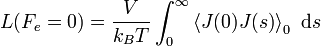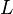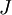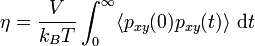# Green-Kubo relationsThis article is a 'stub' page, it has no, or next to no, content. It is here at the moment to help form part of the structure of SklogWiki. If you add sufficient material to this article then please remove the {{Stub-general}} template from this page.

The Green-Kubo relations   are expressions that relate macroscopic transport coefficients to integrals of microscopic time correlation functions. In general one has$L(F_e = 0) =\frac{V}{k_BT} \int_0^\infty \left\langle {J(0)J(s)} \right\rangle _{0} ~{\mathrm{d}} s$

where$L$ is the linear transport coefficient and$J$ is the flux.

## Shear viscosity

The shear viscosity is related to the pressure tensor via$\eta = \frac{V}{k_BT}\int_0^{\infty} \langle p_{xy}(0) p_{xy}(t) \rangle ~{\mathrm{d}} t$

i.e. the integral of the autocorrelation of the off-diagonal components of the microscopic stress tensor.

## Fluctuation theorem

The Green-Kubo relations can be derived from the Evans-Searles transient fluctuation theorem